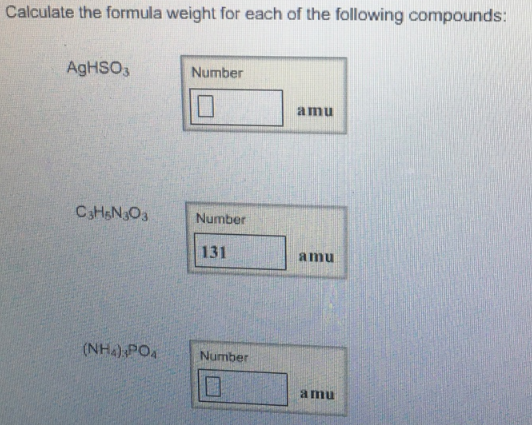# Problem: Calculate the formula weight for each of the following compounds:AgHSO3C3H5N3O3(NH4)3PO4

###### FREE Expert Solution###### Problem Details

Calculate the formula weight for each of the following compounds:

AgHSO3

C3H5N3O3

(NH4)3PO4